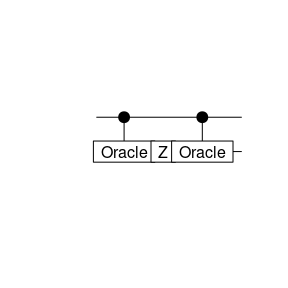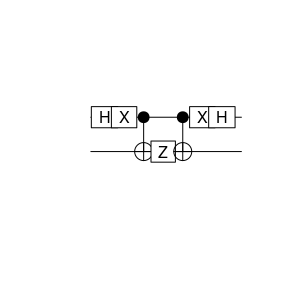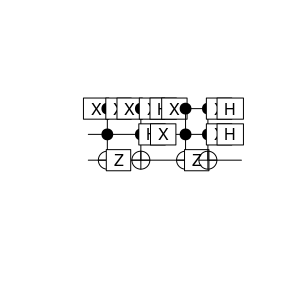# The Algorithm

Grover’s quantum search algorithm is defined via the two following unitary operations $U\ =\ 1-2|x_s\rangle\langle x_s|\,,\quad V\ =\ 1-2|\psi\rangle\langle\psi|\,.$ Here $|\psi\rangle\ =\ \frac{1}{\sqrt{N}}\sum_x |x\rangle\,,$ with states $$|x\rangle$$ in the computational basis and $$N=2^n$$ with $$n$$ the number of qubits. $$x_s$$ is the index of the element sougth for.

The unitary operator $$U$$ is implemented via an oracle function $$f$$ performing the following action $|x\rangle|q\rangle\ \to\ |x\rangle|q\oplus f(x)\rangle$ with $f(x)\ =\ \begin{cases} 1 & x=x_s\,,\\ 0 & \mathrm{ortherwise}\,.\\ \end{cases}$ Thus, the qubit $$q$$ is flipped, if $$f(x)=1$$.

The quantum circuit for $$U$$ looks as followsFor $$V$$ it looks like# Example case $$N=4$$

The case $$n=2$$ and $$N=2^2=4$$ can be implemented as follows: assume $$x_s=2$$, thus we need a function $$f(x) = 1$$ for $$x=2$$ and $$f(x) = 0$$ otherwise. This is achieved as follows:

## oracle for n=2 and x_s=2
oracle <- function(x) {
x <- X(1) * (CCNOT(c(1,2,3)) *(X(1) * x))
return(x)
}

The following test should return 1 only for $$x=x_s$$

## case |00>=0
x <- oracle(qstate(3))
measure(x, 3)$value  0 ## case |01>=1 x <- oracle(X(1)*qstate(3)) measure(x, 3)$value
 0
## case |10>=2
x <- oracle(X(2)*qstate(3))
measure(x, 3)$value  1 ## case |11>=3 x <- oracle(X(2)*(X(1)*qstate(3))) measure(x, 3)$value
 0

The unitaries $$U$$ and $$V$$ for the $$n=2$$ can then be implemented as follows

U <- function(x) {
x <- oracle(x)
x <- Z(3) * x
x <- oracle(x)
return(x)
}
V <- function(x) {
for(i in c(1:2)) {
x <- H(i) * x
}
x <- X(1) * (X(2) * x)
x <- CCNOT(c(1,2,3)) * x
x <- Z(3) * x
x <- CCNOT(c(1,2,3)) * x
x <- X(1) * (X(2) * x)
for(i in c(1:2)) {
x <- H(i) * x
}
return(x)
}

One application of $$V\cdot U$$ looks as follows in the quantum circuit picture$$N=4$$ is the special case where the algorithms returns the correct result with certainty after only a single application of $$V\cdot U$$. This is demonstrated in the following example

## prepare psi
psi <- H(1) * ( H(2) * qstate(3))
## apply VU
x <- U(psi)
x <- V(x)
x
   ( -1 )   * |010> 

As expected, the first two qubits (the two rightmost ones) of x are equal to $$x_s$$ in binary representation. (The phase is not observable.)